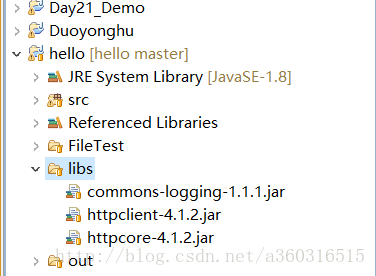Java中Http连接的两种方式(小结)

http协议是基于tcp的一种协议。
tcp是一种保证可靠连接的传输协议，通过三次握手，和丢失重传的机制保证数据的传输。

```String path ="";//
URL url = new URL(path);```

`HttpURLConnection conn = (HttpURLConnection) url.openConnection();`

```conn.setRequestMethod("GET");  //设置本次请求的方式 ， 默认是GET方式， 参数要求都是大写字母
conn.setConnectTimeout(5000);//设置连接超时
conn.setDoInput(true);//是否打开输入流 ， 此方法默认为true
conn.setDoOutput(true);//是否打开输出流， 此方法默认为false
conn.connect();//表示连接```

`int code = conn.getResponseCode();`

```  InputStream is = conn.getInputStream();

String name = path.substring(path.lastIndexOf("/")+1);

System.out.println("name = " + name);

fos = new FileOutputStream("C:\\pro\\"+name);

byte[] buffer = new byte;
int len = 0;

fos.write(buffer, 0, len);```

```OutputStream os = conn.getOutputStream();
os.write("platform=2&appVersion=1.7.0&osType=2".getBytes());
os.flush();```

HttpClient??

`String path = "";`

`HttpClient client = new DefaultHttpClient();`

`HttpGet httpGet = new HttpGet(path);`

`HttpResponse response = client.execute(httpGet);`

`response.getStatusLine().getStatusCode() == 200;`

`HttpEntity entity = response.getEntity();`

Post方式

```HttpPost httpPost = new HttpPost(path);

//创建一个提交数据的容器
List<BasicNameValuePair> parames = new ArrayList<>();

//封装容器到请求参数中
HttpEntity entity = new UrlEncodedFormEntity(parames);
//设置请求参数到post请求中
httpPost.setEntity(entity);

//执行post请求
HttpResponse response = client.execute(httpPost);```